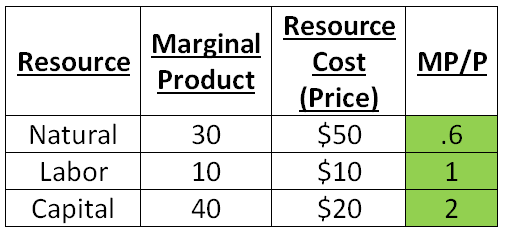### ReviewEcon.com

AP, IB, and College Microeconomic and Macroeconomic Principles

# Least Cost Combinations and Profit Maximizing Combinations

Updated 12/15/2016 Jacob Reed
Quickly review profit maximizing and least cost combinations of resources then practice with a review game at the bottom. The first five questions are about utility maximizing combinations, and the second five questions are about profit maximizing and least cost combinations.Diminishing Marginal Returns tells us that as a firm hires more units of a resource, the marginal product of those resources eventually diminishes.

Marginal Product per Dollar is a calculation of a marginal product to cost ratio for a firm’s hiring of an additional unit of a resource. The formula is marginal product divided by price of the resource (MP/P). So if the last worker hired has a marginal product of 15 and that worker costs \$5, the marginal product per dollar is 3.

Marginal Revenue Product per Dollar is a calculation of the marginal revenue product to cost ratio for a firm’s hiring of an additional unit of a resource. The formula is marginal revenue product divided by price of the resource (MRP/P). Using the example above, if each unit sells for \$10 then the last worker has a marginal revenue product of \$150 (15x\$10). That means the marginal revenue product per dollar of the last worker hired is \$30 (\$150/\$5)

Least Cost Combination occurs when a firm adjusts their employment of resources to minimize costs. The least cost combination is found where the marginal product per dollar for all the resources a firm employs are equal (MPL/PL=MPN/PN=MPC/PC). If the ratios are not equal, a firm would reduce cost by employing more of the resource with a higher MP/P and less of the resource with a lower MP/P.In the example above, the firm should employ more Capital and fewer Natural Resources.

Profit Maximizing Combination is exactly the same as least cost, except that marginal revenue product per dollar is used instead of marginal product per dollar. So the formula profit maximizing combination is (MRPL/PL=MRPN/PN=MRPC/PC).

Note: These resource combinations are very similar to utility maximization for consumers with combinations of goods and services.

Multiple Choice Connections:
2012 Released AP Microeconomics Exam Questions: 43

Up Next:
Review Game: Product and Resource Combinations
Content Review Page: Externalities

Other recommended resourcejsearcysfc